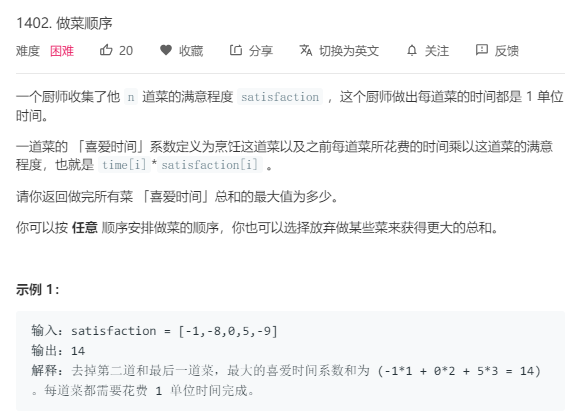1402.做菜顺序https://leetcode-cn.com/problems/reducing-dishes/solution/tan-xin-dong-tai-gui-hua-by-xjtupanda/

dp[i]表示花费i时间可以获得的最大值

class Solution {
public:
int dp;
int maxSatisfaction(vector<int>& satisfaction) {
memset(dp,0x80,sizeof(dp));
sort(satisfaction.begin(),satisfaction.end());
dp = 0;
int n = satisfaction.size();
for(int i=0;i<n;i++){
for(int j=i;j>=0;j--){
dp[j+1] = max(dp[j+1], satisfaction[i]*(j+1)+dp[j]);
}
}
int ans = 0;
for(int i=1;i<=n;i++)ans = max(ans,dp[i]);
return ans;
}
};


1